Transfer Function Stability - Interesting Facts about Polynomial Form

### Transfer Function Stability - Interesting Facts about Polynomial Form

Transfer function stability is solely determined by its denominator. The roots of a denominator are called poles.

Poles located in the left half-plane are stable while poles located in the right half-plane are not stable.

The reasoning is very simple: the Laplace operator "s", which is location in the Laplace domain, can be also written as: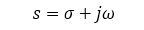Left half-plane has negative sigma.

For a first-order system, the following transfer function corresponds to this time domain differential equation: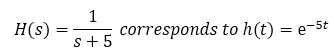Consider a step change as the input: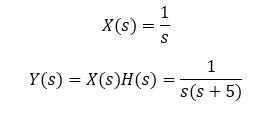Hence: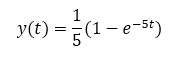The output converges to 0.2, a steady-state and stable value.

Generally, consider the following generic denominator: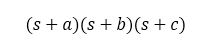If such a polynomial is expanded and terms are collected, we obtain: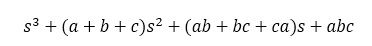Claim: If all polynomial coefficients are positive, all roots are negative and the system is stable

In short:

• If all polynomial coefficients are positive, then all poles are negative.
• If all roots are negative, then all polynomial coefficients are positive.

If all three terms a, b, and c are positive, then all three poles are located in the left-half plane and the system is stable. Also, notice that all coefficient of the expanded polynomial are positive.

E.g., a = 7, b = 2, c = 4, (poles are located at -7, -2, and -4).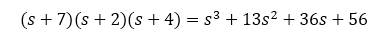For a polynomial containing complex poles as shown below, the imaginary part sign does not matter since there needs to be the complex pole counterpart.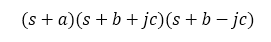Thus, it suffices that a and b are positive: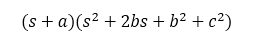How do we know that the second polynomial (s2+2bs+b2+c2) has negative roots?

The b2 and c2 terms are always positive and the b term is positive as indicated above.

Therefore, if all coefficients are positive, all roots are negative and the system is stable.

Claim: If any polynomial coefficient is negative, at least one root is positive and the system is unstable

Without a proof here.

Claim: If a polynomial coefficient is zero, at least one root is positive and the system is unstable

See the following polynomial: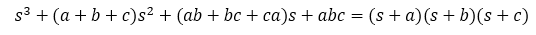Case 1: s2(a+b+c) = 0

This means that at least one term (from a, b, c) is negative, otherwise the sum would be positive.

Case 2: s(ab+bc+ca) = 0

From the way the coefficient is structured, at least one term has to be negative or all three terms need to be zero otherwise the coefficient would be positive.

Case 3: abc = 0

This term can be zero. This also means that at least one root is zero as well.

e.g. a = 0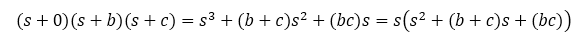Major takeaway: based on this analysis (by no means a complete proof) when a coefficient of a power of s is zero, then the system is unstable.

Example: A quick stability analysis of a closed-loop system

Consider the following system. For which gain Kp does the closed-loop system becomes stable?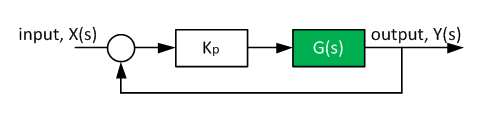The plant transfer function G(s) is shown below: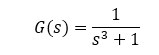The complete closed-loop transfer function, including the proportional controller Kp, is: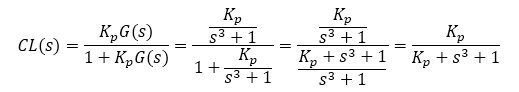Answer: No proportional controller gain can make the system stable since the closed-loop transfer function has zero "s" and "s2" term coefficients. All coefficients of "sn" must be positive.

Root locus follows (notice the two right half-plane poles).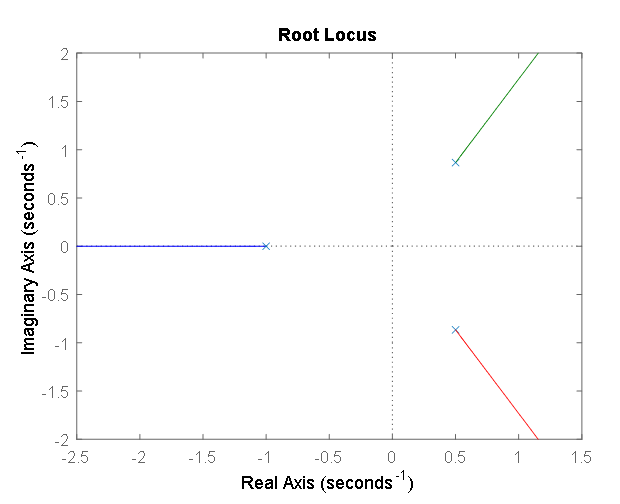Version

Author(s)

TS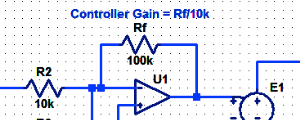#### Proportional Controller Implementation

In MatLab, DSPs, and FPGAs.

.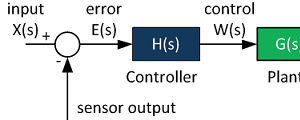#### Control System Block Diagram

The fundamentals of signal flow.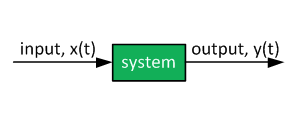#### System Modeling With Transfer Functions

Introduction to dynamic systems.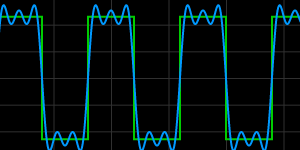#### Fourier Series Demo

It is all sine waves.